# Household ElectricityPage 1

#### WATCH ALL SLIDES

Slide 1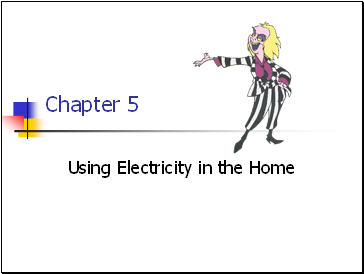Chapter 5

Using Electricity in the Home

Slide 2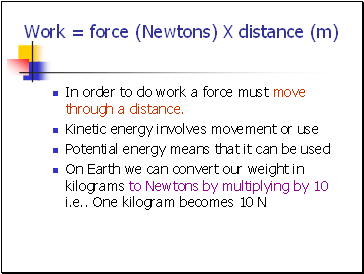## Work = force (Newtons) X distance (m)

In order to do work a force must move through a distance.

Kinetic energy involves movement or use

Potential energy means that it can be used

On Earth we can convert our weight in kilograms to Newtons by multiplying by 10 i.e One kilogram becomes 10 N

Slide 3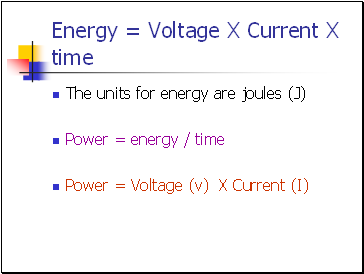Energy = Voltage X Current X time

The units for energy are joules (J)

Power = energy / time

Power = Voltage (v) X Current (I)

Slide 4## House Hold Energy Consumption

Hydro bills are established by finding out how many kilowatt hours of electricity your household has used in a given time.

1 kWh = kilowatts X time (hours)

1 kWh = 1 000 Watts X 1 hour

1 kWh = 1 000 W X 3 600 seconds

Slide 5Reading the Meter – always use the smallest number

Slide 6Slide 7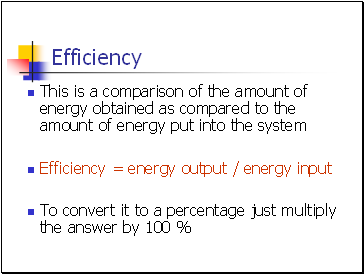## Efficiency

This is a comparison of the amount of energy obtained as compared to the amount of energy put into the system

Efficiency = energy output / energy input

To convert it to a percentage just multiply the answer by 100 %

Slide 8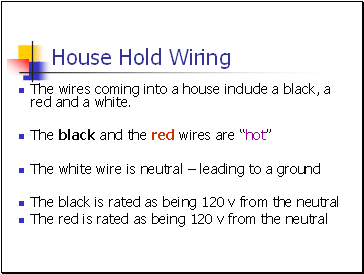## House Hold Wiring

The wires coming into a house include a black, a red and a white.

The black and the red wires are “hot”

The white wire is neutral – leading to a ground

The black is rated as being 120 v from the neutral

The red is rated as being 120 v from the neutral

Slide 9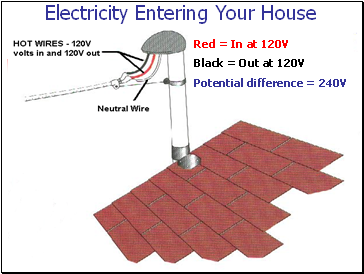Red = In at 120V

Black = Out at 120V

Potential difference = 240V

Slide 10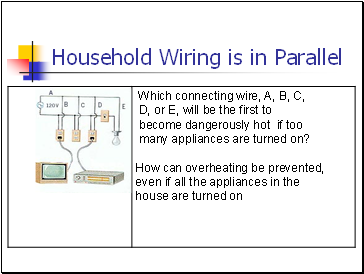## Household Wiring is in Parallel

Slide 11## Alternating Current Frequency

In North America, the current alternates back and forth in what we call cycles per second

1 cycle/second = 1 Hertz

Electrical frequency in North America = 60 Hz

Slide 12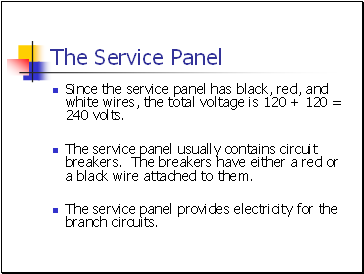Go to page:
1  2  3